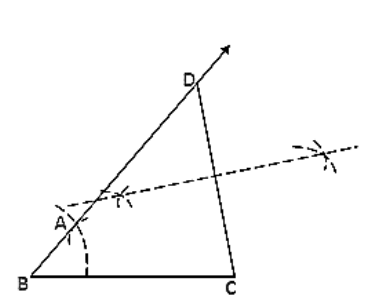# Construct a △ABC in which BC = 3.6 cm, AB + AC = 4.8 cm and ∠B = 60°.

Question:

Construct a △ABC in which BC = 3.6 cm, AB + AC = 4.8 cm and ∠B = 60°.

Solution:

Steps of Construction:

1. Construct a line segment BC of 3.6 cm.

2. At the point B, draw ∠XBC = 60°.

3. Keeping B as center and radius 4.8cm draw an arc which intersects XB at D.

4. Join DC.

5. Draw the perpendicular bisector of DC which intersects DB at A.

6. Join AC.

Hence △ABC is the required triangle.